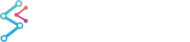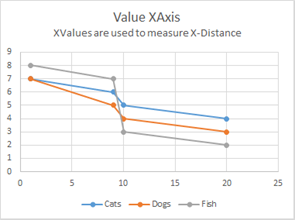﻿ Value Axis vs. Category Axis | WPF Chart DocumentationSciChart WPF 2D Charts > Axis APIs > Value Axis vs. Category Axis
Value Axis vs. Category Axis

SciChart 2D WPF Features several axis types. All inherit from AxisBase.

## What is a Value Axis vs. a Category Axis?

SciChart WPF Features both Value Axis and Category Axis types. An explanation of these is given below:

Given the data

 Age (XAxis) Cats Dogs Fish 1 7 7 8 9 6 5 7 10 5 4 3 20 4 3 2

A Value X-Axis and Category X-Axis would display the data differently:The fundamental difference is a Value-Axis uses the X-Value to compute distance on the XAxis, whereas a Category-Axis uses the X-Index. Value-Axis are primarily used in scientific software where Category –Axis are typically used in financial software (e.g. stock charts).

SciChart features both Value-Axis and Category-Axis types.

 Axis Type Value or Category Axis NumericAxis Value Axis LogarithmicNumericAxis Value Axis DateTimeAxis Value Axis TimeSpanAxis Value Axis CategoryDateTimeAxis Category Axis